### Example 71.8 Survival Curves

You might want to use your regression analysis results to predict the survivorship of subjects of specific covariate values. The COVARIATES= data set in the BASELINE statement enables you to specify the sets of covariate values for the prediction. On the other hand, you might want to summarize the survival experience of an average patient for a given population. The DIRADJ option in the BASELINE statement computes the direct adjusted survival curve that averages the estimated survival curves for patients whose covariates are represented in the COVARIATES= data set. By using the PLOTS= option in the PROC PHREG statement, you can use ODS Graphics to display the predicted survival curves. You can elect to output the predicted survival curves in a SAS data set by optionally specifying the OUT= option in the BASELINE statement. This example illustrates how to obtain the covariate-specific survival curves and the direct adjusted survival curve by using the Myeloma data set in Example 71.1, where variables LogBUN and HGB were identified as the most important prognostic factors.

Suppose you want to compute the predicted survival curves for two sets of covariate values: (LogBUN=1.0, HGB=10) and (LogBUN=1.8, HGB=12). These values are saved in the data set Inrisks in the following DATA step. Also created in this data set is the variable Id, whose values will be used in identifying the covariate sets in the survival plot.

data Inrisks;
length Id $20; input LogBUN HGB Id$12-31;
datalines;
1.00 10.0  logBUN=1.0 HGB=10
1.80 12.0  logBUN=1.8 HGB=12
;


The following statements plot the survival functions in Output 71.8.1 and save the survival estimates in the data set Pred1:

ods graphics on;
proc phreg data=Myeloma plots(overlay)=survival;
model Time*VStatus(0)=LogBUN HGB;
baseline covariates=Inrisks out=Pred1 survival=_all_/rowid=Id;
run;


The COVARIATES= option in the BASELINE statement specifies the data set that contains the set of covariates of interest. The PLOTS= option in the PROC PHREG statement creates the survival plot. The OVERLAY suboption overlays the two curves in the same plot. If the OVERLAY suboption is not specified, each curve is displayed in a separate plot. The ROWID= option in the BASELINE statement specifies that the values of the variable Id in the COVARIATES= data set be used to identify the curves in the plot. The SURVIVAL=_ALL_ option in the BASELINE statement requests that the estimated survivor function, standard error, and lower and upper confidence limits for the survivor function be output into the SAS data set that is specified in the OUT= option.

The survival Plot (Output 71.8.1) contains two curves, one for each of row of covariates in the data set Inrisks.

Output 71.8.1: Estimated Survivor Function Plot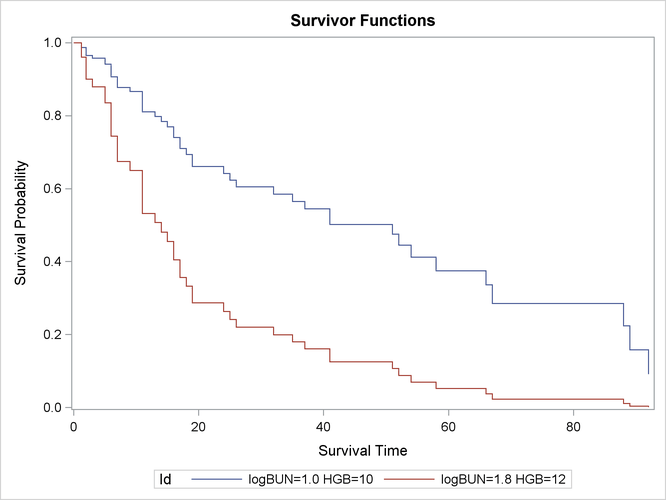The following statements print out the observations in the data set Pred1 for the realization LogBUN=1.00 and HGB=10.0:

proc print data=Pred1(where=(logBUN=1 and HGB=10));
run;


As shown in Output 71.8.2, 32 observations represent the survivor function for the realization LogBUN=1.00 and HGB=10.0. The first observation has survival time 0 and survivor function estimate 1.0. Each of the remaining 31 observations represents a distinct event time in the input data set Myeloma. These observations are presented in ascending order of the event times. Note that all the variables in the COVARIATES=InRisks data set are included in the OUT=Pred1 data set. Likewise, you can print out the observations that represent the survivor function for the realization LogBUN=1.80 and HGB=12.0.

Output 71.8.2: Survivor Function Estimates for LogBUN=1.0 and HGB=10.0

Obs Id LogBUN HGB Time Survival StdErrSurvival LowerSurvival UpperSurvival
1 logBUN=1.0 HGB=10 1 10 0.00 1.00000 . . .
2 logBUN=1.0 HGB=10 1 10 1.25 0.98678 0.01043 0.96655 1.00000
3 logBUN=1.0 HGB=10 1 10 2.00 0.96559 0.01907 0.92892 1.00000
4 logBUN=1.0 HGB=10 1 10 3.00 0.95818 0.02180 0.91638 1.00000
5 logBUN=1.0 HGB=10 1 10 5.00 0.94188 0.02747 0.88955 0.99729
6 logBUN=1.0 HGB=10 1 10 6.00 0.90635 0.03796 0.83492 0.98389
7 logBUN=1.0 HGB=10 1 10 7.00 0.87742 0.04535 0.79290 0.97096
8 logBUN=1.0 HGB=10 1 10 9.00 0.86646 0.04801 0.77729 0.96585
9 logBUN=1.0 HGB=10 1 10 11.00 0.81084 0.05976 0.70178 0.93686
10 logBUN=1.0 HGB=10 1 10 13.00 0.79800 0.06238 0.68464 0.93012
11 logBUN=1.0 HGB=10 1 10 14.00 0.78384 0.06515 0.66601 0.92251
12 logBUN=1.0 HGB=10 1 10 15.00 0.76965 0.06779 0.64762 0.91467
13 logBUN=1.0 HGB=10 1 10 16.00 0.74071 0.07269 0.61110 0.89781
14 logBUN=1.0 HGB=10 1 10 17.00 0.71005 0.07760 0.57315 0.87966
15 logBUN=1.0 HGB=10 1 10 18.00 0.69392 0.07998 0.55360 0.86980
16 logBUN=1.0 HGB=10 1 10 19.00 0.66062 0.08442 0.51425 0.84865
17 logBUN=1.0 HGB=10 1 10 24.00 0.64210 0.08691 0.49248 0.83717
18 logBUN=1.0 HGB=10 1 10 25.00 0.62360 0.08921 0.47112 0.82542
19 logBUN=1.0 HGB=10 1 10 26.00 0.60523 0.09136 0.45023 0.81359
20 logBUN=1.0 HGB=10 1 10 32.00 0.58549 0.09371 0.42784 0.80122
21 logBUN=1.0 HGB=10 1 10 35.00 0.56534 0.09593 0.40539 0.78840
22 logBUN=1.0 HGB=10 1 10 37.00 0.54465 0.09816 0.38257 0.77542
23 logBUN=1.0 HGB=10 1 10 41.00 0.50178 0.10166 0.33733 0.74639
24 logBUN=1.0 HGB=10 1 10 51.00 0.47546 0.10368 0.31009 0.72901
25 logBUN=1.0 HGB=10 1 10 52.00 0.44510 0.10522 0.28006 0.70741
26 logBUN=1.0 HGB=10 1 10 54.00 0.41266 0.10689 0.24837 0.68560
27 logBUN=1.0 HGB=10 1 10 58.00 0.37465 0.10891 0.21192 0.66232
28 logBUN=1.0 HGB=10 1 10 66.00 0.33626 0.10980 0.17731 0.63772
29 logBUN=1.0 HGB=10 1 10 67.00 0.28529 0.11029 0.13372 0.60864
30 logBUN=1.0 HGB=10 1 10 88.00 0.22412 0.10928 0.08619 0.58282
31 logBUN=1.0 HGB=10 1 10 89.00 0.15864 0.10317 0.04435 0.56750
32 logBUN=1.0 HGB=10 1 10 92.00 0.09180 0.08545 0.01481 0.56907

Next, the DIRADJ option in the BASELINE statement is used to request a survival curve that represents the survival experience of an average patient in the population in which the COVARIATES= data set is sampled. When the DIRADJ option is specified, PROC PHREG computes the direct adjusted survival function by averaging the predicted survival functions for the rows in the COVARIATES= data set. The following statements plot the direct adjusted survival function in Output 71.8.3.

proc phreg data=Myeloma plots=survival;
model Time*VStatus(0)=LogBUN HGB;
run;


When the DIRADJ option is specified in the BASELINE statement, the default COVARIATES= data set is the input data set. For clarity, the COVARIATES=MYELOMA is specified in the BASELINE statement in the preceding PROC PHREG call.

Output 71.8.3: Average Survival Function for the Myeloma Data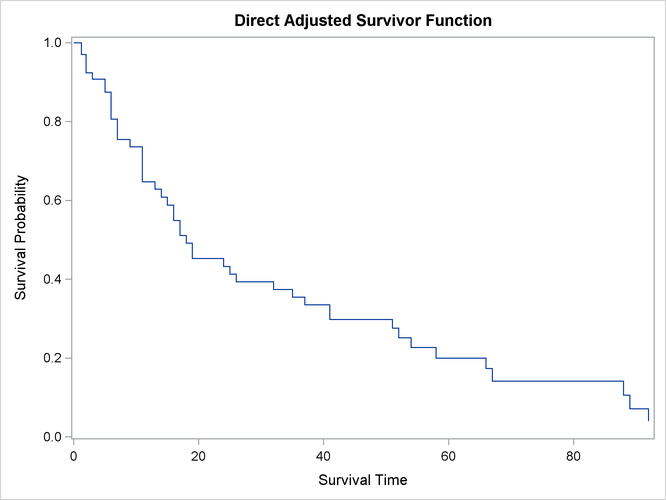If neither the COVARIATES= data set nor the DIRADJ option is specified in the BASELINE statement, PROC PHREG computes a predicted survival curve based on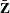, the average values of the covariate vectors in the input data (Neuberger et al., 1986). This curve represents the survival experience of a patient with an average prognostic index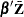equal to the average prognostic index of all patients. This approach has a couple of drawbacks: it is possible that no patient could ever have such an average index, and it does not account for the variability in the prognostic factor from patient to patient.

The DIRADJ option is particularly useful if the model contains a categorical explanatory variable that represents different treatments of interest. By specifying this categorical variable in the GROUP= option, you obtain a direct adjusted survival curve for each category of the variable. In addition, you can use the OUTDIFF= option to save all pairwise differences of these direct adjusted survival probabilities in a data set. For illustration, consider a model that also includes the categorical variable Frac, which has a value 0 if a patient did not have a fracture at diagnosis and 1 otherwise, as an explanatory variable. The following statements plot the adjusted survival curves in Output 71.8.4 and save the differences of the direct adjusted survival probabilities in the data set Diff1:

proc phreg data=Myeloma plots(overlay)=survival;
class Frac;
model Time*VStatus(0)=LogBUN HGB Frac;
baseline covariates=Myeloma outdiff=Diff1 survival=_all_/diradj group=Frac;
run;


Because the CLASS variable Frac is specified as the GROUP= variable, a separate direct adjusted survival curve is computed for each value of the variable Frac. Each direct adjusted survival curve is the average of the predicted survival curves for all the patients in the entire Myeloma data set with their Frac value set to a specific constant. For example, the direct adjusted survival curve for Frac=0 (no fracture at diagnosis) is computed as follows:

1. The value of the variable Frac is set to 0 for all observations in the Myeloma data set.

2. The survival curve for each observation in the modified data set is computed.

3. All the survival curves computed in step 2 are averaged.

Output 71.8.4: Average Survival by Fracture Status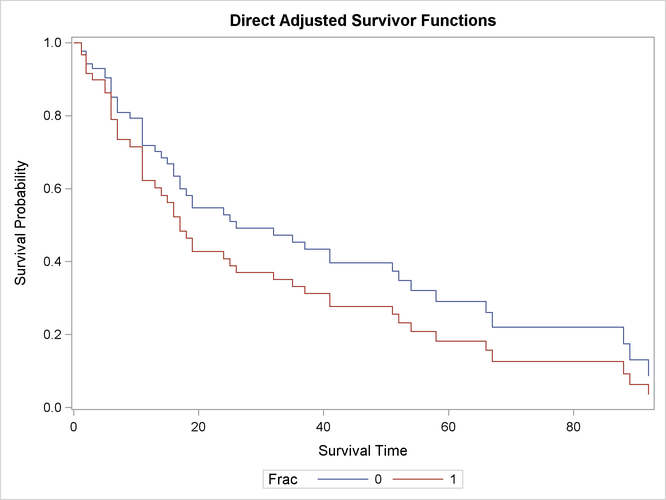Output 71.8.4 shows that patients without fracture at diagnosis have better survival than those with fractures. Differences in the survival probabilities and their standard errors are displayed in Output 71.8.5.

proc print data=Diff1;
run;


Output 71.8.5: Differences in the Survival between Fracture and Nonfracture

Obs Frac Frac2 Time SurvDiff StdErr
1 0 1 1.25 0.01074 0.01199
2 0 1 2.00 0.02653 0.02605
3 0 1 3.00 0.03165 0.03063
4 0 1 5.00 0.04191 0.03963
5 0 1 6.00 0.06115 0.05669
6 0 1 7.00 0.07416 0.06853
7 0 1 9.00 0.07854 0.07261
8 0 1 11.00 0.09669 0.09002
9 0 1 13.00 0.09998 0.09327
10 0 1 14.00 0.10319 0.09644
11 0 1 15.00 0.10611 0.09937
12 0 1 16.00 0.11117 0.10464
13 0 1 17.00 0.11532 0.10922
14 0 1 18.00 0.11704 0.11120
15 0 1 19.00 0.11969 0.11447
16 0 1 24.00 0.12072 0.11593
17 0 1 25.00 0.12145 0.11713
18 0 1 26.00 0.12189 0.11808
19 0 1 32.00 0.12208 0.11883
20 0 1 35.00 0.12197 0.11933
21 0 1 37.00 0.12155 0.11956
22 0 1 41.00 0.11983 0.11924
23 0 1 51.00 0.11821 0.11850
24 0 1 52.00 0.11580 0.11714
25 0 1 54.00 0.11262 0.11507
26 0 1 58.00 0.10824 0.11203
27 0 1 66.00 0.10301 0.10814
28 0 1 67.00 0.09451 0.10130
29 0 1 88.00 0.08248 0.09133
30 0 1 89.00 0.06847 0.08033
31 0 1 92.00 0.05038 0.06515